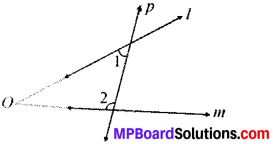# MP Board Class 9th Maths Solutions Chapter 5 Introduction to Euclid’s Geometry Ex  5.2

## MP Board Class 9th Maths Solutions Chapter 5 Introduction to Euclid’s Geometry Ex  5.2Question 1.
How would you rewrite Euclid’s fifth postulate so that it would be easier to understand?
Solution:
If a line p intersects two lines l and m such that (∠1 + ∠2) is less than 180°, then lines l and m will meet at O, as shown in Fig. below.Question 2.
Does Euclid’s fifth postulate imply the existence of parallel lines? Explain.
Solution:
Yes, Euclid’s fifth postulate is important to express parallel lines. Two lines will never meet if they are not according to Euclid’s fifth postulate.